Click the {…} button to add code to your post: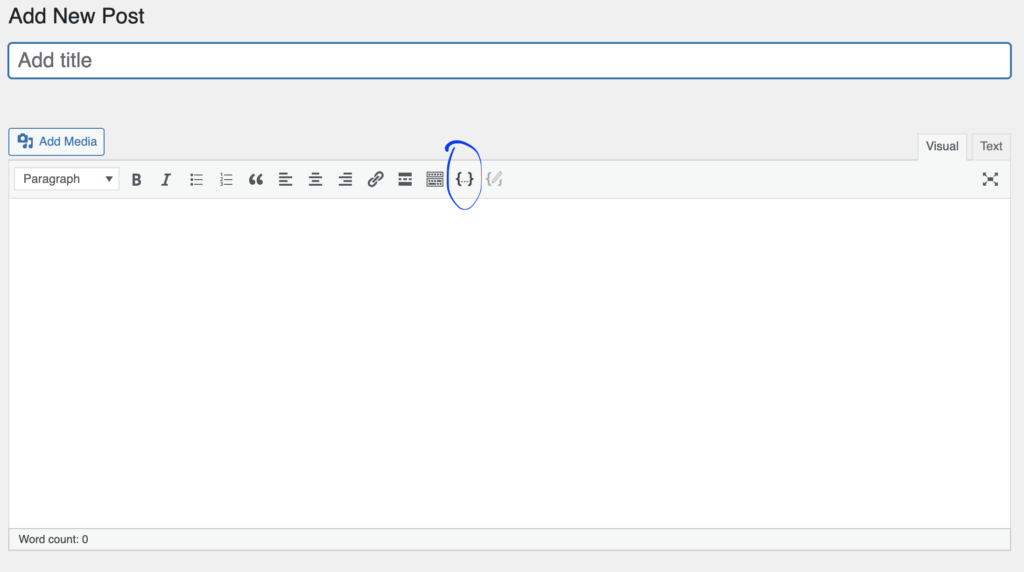You’ll then see this screen where you can paste your code in: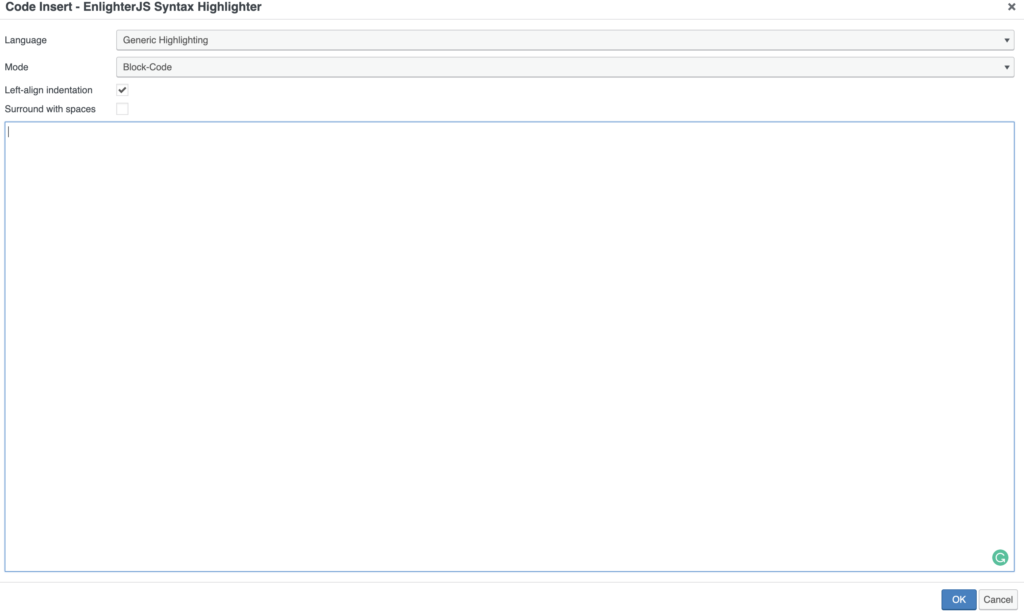For Processing code change the language to Java: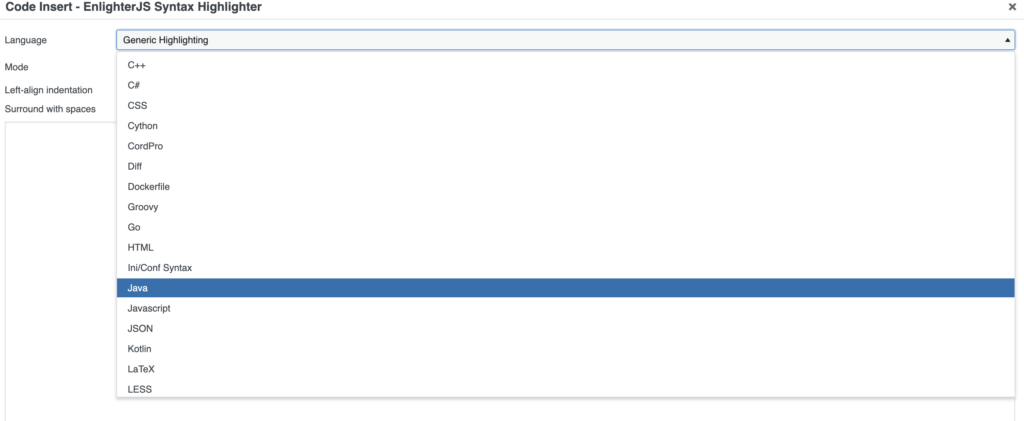Once you’re done click Add and you’re all set. Easy!

```int rectWidth = 8;

void setup() {
size(640, 480);
rectMode(CENTER);
}

void draw() {
background(255);
for (int x = 0; x<width; x+=rectWidth) {
float center = height*.25;
float amplitude = 100;
float speed = .01;
float granular = .001;
float freq = (frameCount* speed) + (x * granular);
float adjustedHeight = noise(freq);// between 0 & 1
adjustedHeight -= .5;// between -0.5 & 0.5
rect(x + rectWidth/2, center + adjustedHeight, rectWidth, 100);
}
for (int x = 0; x<width; x+=rectWidth) {
float center = height - (height*.25);
float amplitude = TWO_PI;
float speed = .01;
float granular = .001;
float freq = (frameCount* speed) + (x * granular);
float angle = noise(freq);// between 0 & 1
angle *= amplitude; // between 0 & TWO_PI
pushMatrix();
translate(x + rectWidth/2, center);
rotate(angle);
rect(0,0, rectWidth, 100);
popMatrix();
}
}```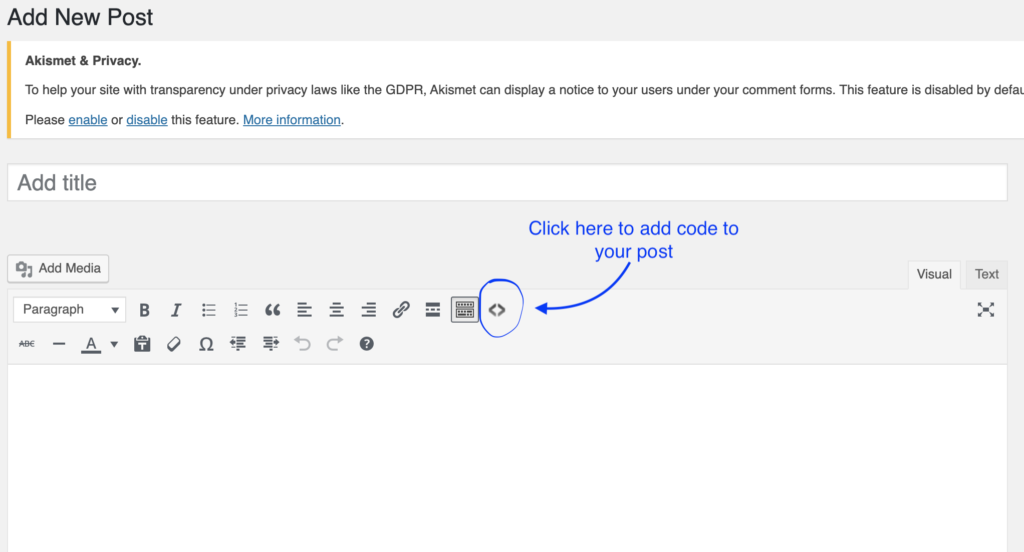You’ll then see this screen where you can paste your code in: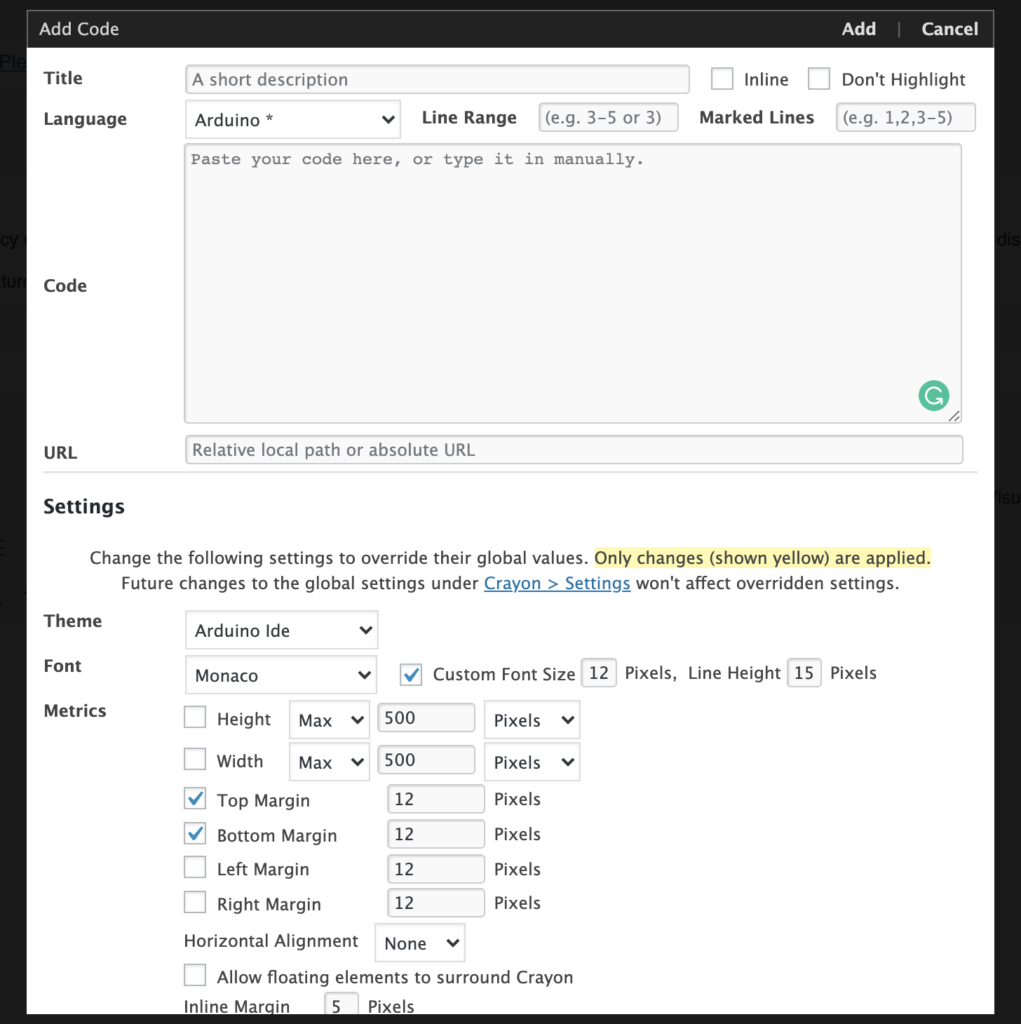For Processing code change the language to Java: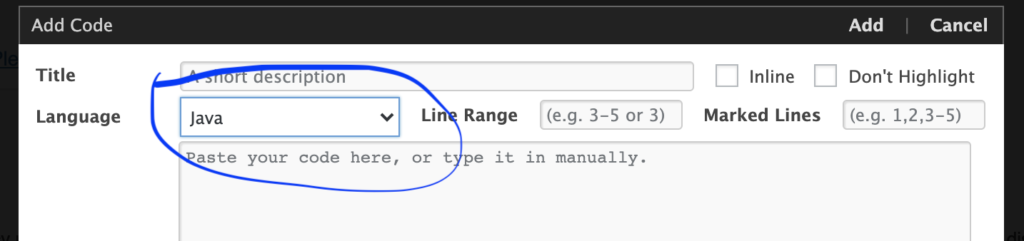Once you’re done click Add and you’re all set. Easy!

```int x;
int y;
int backgroundColor = 255;
float r = 255;
float g = 0;
float b = 0;
int rectWidth = 100;
int rectHeight = 100;

void setup() {
size(640, 480);
x = width/2;
y = height/2;
}

void draw() {
background(backgroundColor);
noStroke();
fill(r, g, b);
rect(x, y, rectWidth, rectHeight);
}

void mousePressed() {
if (mouseX > x && mouseX < x+rectWidth && mouseY > y && mouseY < y+rectHeight) {
r = random(255);
g = random(255);
b = random(255);
}
}

void keyPressed() {
if (key=='b') {
backgroundColor = 255 - backgroundColor;
}
}```